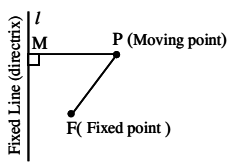# Definition of a Conic

The curves obtained by slicing the cone with a plane not passing through the vertex are called conic sections or conics.

A conic is the locus of a point which moves in a plane, so that its distance from a fixed point bears a constant ratio to its distance from a fixed straight line.

The fixed point is called focus, the fixed straight line is called directrix, and the constant ratio is called eccentricity.FP/PM = e